List price prediction with scraped real-estate data

This is the first of two articles about using data scraped from the web and machine learning to predict the list price of real-estate listings.

This first article focuses on a tabular set of numerical and categorical features we collected describing the listings. We will explore these features and show how they can be processed and used in a supervised regression model to predict the list price.

In the second article, we will focus on a textual description variable we collected and see how we can leverage the latest advances in NLP to achieve similar or better list price prediction performances. Through this practical example, we will be showing how BERT can be fine-tuned for a regression task.

The data set

The data set used in this article was scrapped from French real-estate websites.

This dataset is different from usual housing datasets since it focuses on list prices and not transaction prices. We can expect some bias between the two, list prices are expected to be on average higher than effective transaction prices. The biggest advantage of this dataset is that we have many features that are often absent from housing datasets.

It includes data from 788K listings such as the one below for which 31 features were collected.

The features we have at hand are:

• the list price which will be our target variable,
• the property type (house, apartment, condo, mansion…),
• the location (latitude, longitude, city, department),
• the size (living area and land area when applicable),
• the number of rooms, bedrooms, bathrooms…
• energy performance indicators (energy and greenhouse gas emissions)
• the number of photos attached to the listing,
• indicators whether there is a garden, a balcony, air conditioning…
• and lastly, a free text form description of the property in French. We will talk more about this textual feature and NLP in the second article.

Feature exploration

Train test split

The exploratory data analysis is expected to influence our pre-processing and modelling decisions. To minimize our bias when evaluating performance later, we will do a train test split straight away and focus on the training set.

import pandas as pd
from sklearn.model_selection import train_test_split
file_path = "real_estate_data.csv"
train_data, test_data = train_test_split(data, train_size=int(3e5),
random_state=42)
df = train_data

The data set we are dealing is voluminous, in it’s original CSV file format it takes up 1.68GB. Training complex machine learning models on such large data with limited computing resources will be time consuming. For this reason, we will only consider 300K observations for training out of the original 788K. The rest will be kept for evaluation purposes. Thus, we assume that the random sample of ~40% taken from our set is representative of the problem domain.

Duplicate values

With this one-liner, we can confirm that none of the rows are exact duplicates of each other across all features.

df.duplicated(keep = False).sum()
# returns 0

That said, certain features alone should not contain any duplicate values by nature. This is the case for the listing ID (“id_annonce” variable) and arguably for the textual description of the listing (“description” variable).

It turns out 1.25% of the rows have duplicate listing IDs and 21% have duplicate descriptions. Since none of these rows are duplicates of each other across all features we looked into which features tend to differ for ID or description duplicates.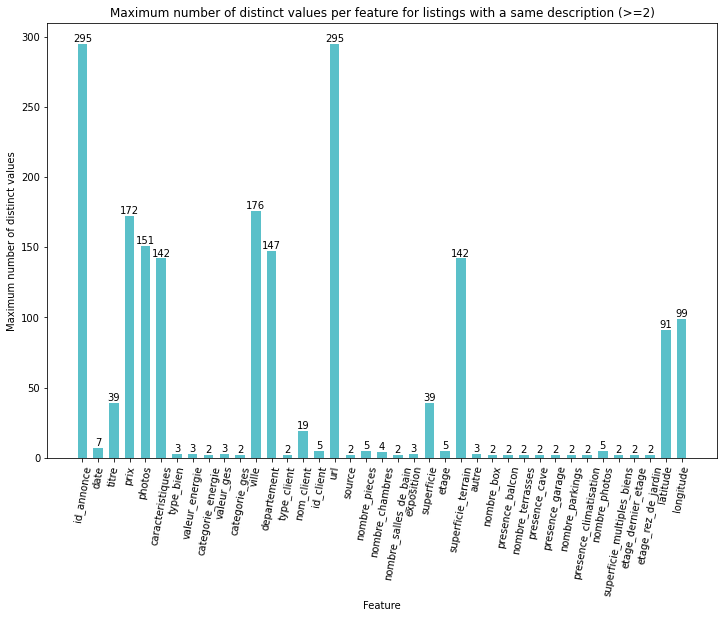Number of distinct values for listings with duplicate IDs or descriptions (only displaying values ≥ 2)

Having listings with a same ID but a different price (“prix”), number of rooms (“nombre_pieces”), size (“superficie”)… seems incoherent. Likewise, we would not expect properties with a same description to have a different ID, price, number of rooms (“nombre_chambres”)… These duplicates are thus removed from the set altogether.

df.drop_duplicates(subset = "id_annonce", keep=False, inplace=True)
df.drop_duplicates(subset = "description", keep=False, inplace=True)

Removing these duplicates reduces the size of the training set by 21.12%.

Missing values

Missing value rates in our features can quickly be calculated with the following line of code:

df.isna().sum()/len(df)

To avoid using artificial values in our target variable, any row where the price is missing is discarded.

To guarantee coherent geographical analyses later on and since there are only few, rows with missing latitude and longitude values are also removed.

df = df.dropna(subset = ['prix', 'latitude', 'longitude'])

Part of the missing values in living area (“superficie”) and land size (“superficie_terrain”) can be imputed based on the type of property (“type_bien”). We can assume that appartements (“appartement”), lofts and single rooms (“chambre”) have a land size of zero. Likewise, we can assume that plots of land or building sites (“terrain” and “terrain à bâtir”) have a living area of zero.

df.loc[df.type_bien.isin(['appartement', 'loft', 'chambre']) \
& df.superficie_terrain.isna(), 'superficie_terrain'] = 0
rows = df.type_bien.isin(['terrain à bâtir', 'terrain']) \
& df.superficie_terrain.isna() \
& ~df.superficie.isna()
df.loc[rows, 'superficie_terrain'] = df.loc[rows, 'superficie']
df.loc[rows, 'superficie'] = 0

Doing so, the missing value rate in the living area slightly decreases while in the land size variable it drops from 40% to 9%.

The energy performance variables (features ending by “_energie” or “_ges”) go by pairs. One is a numerical value (starting with “valeur_”), the other (starting with “categorie_”) is a category “A”, “B”, “C”… based on the latter numerical value. Missing energy performance variables could have been imputed based on the observed value-category correspondence but unfortunately, when one is missing, the other is missing too. It can be visualised using the missingno toolset.

import missingnomissingno.matrix(df[['categorie_energie', 'valeur_energie',
'categorie_ges', 'valeur_ges']])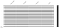Missingno matrix displaying missing observations in a feature as black lines for the energy performance variables

Missing values in the rest of the features, including the energy performance variables, are thus imputed by default with the median value for numerical features and an “Unknown” modality for categorical ones.

from pandas.api.types import is_string_dtype
from pandas.api.types import is_numeric_dtype
for col in df.columns:
if col != 'prix':
if is_numeric_dtype(df[col]):
df[col] = df[col].fillna(df[col].median())
elif is_string_dtype(df[col]):
df[col] = df[col].fillna('Unknown')

After dealing with duplicates and missing values, the training data set is 21.4% smaller than the original 300K observations, standing at 235K observations.

Outliers

Looking into the distributions of the features, we notice that some values are incoherent or problematic for our price prediction objective.

Continuous numerical features

Focusing on continuous numerical variables, we notice that the distribution of the top 1% of the price, energy performance, greenhouse gas emission, living and land size are far off from the remaining 99% of the values. The maximum value for these features is 10e3 to 10e6 times greater than the 99th percentile. Most of these outliers seem incoherent by nature. They are also likely to be problematic when modelling or evaluating performances, especially for the outliers in the target variable.

continuous_numerical = [
'prix', # price
'valeur_energie', # energy performance value
'valeur_ges', # greenhouse gas emission performance value
'latitude',
'longitude',
'superficie', # living area
'superficie_terrain', # land size
]
df[continuous_numerical].describe([0.1, 0.25, 0.5, 0.75, 0.9,
0.99]).round()

Discrete numerical features

Likewise, focusing on the discrete numerical values, we notice that the maximum value is an order of magnitude of 10 to 100 times greater than the 99th percentile for most of these features.

discrete_numerical = [
'nombre_pieces', #number of rooms
'nombre_chambres', #number of bedrooms
'nombre_salles_de_bain', #number of bathrooms
'etage', #floor
'nombre_box', #number of boxes
'nombre_terrasses', #number of terrasses
'nombre_parkings', #number of parking spots
'nombre_photos'#number of photos attached to the listing
]
df[discrete_numerical].describe([0.1, 0.25, 0.5, 0.75, 0.9, 0.99]).round()

Outlier removal in numerical features

The top 1% of the continuous and discrete numerical features with outliers is thus removed from the set. The bottom 1% of the price feature is also deemed incoherent and is discarded from the set.

def remove_outliers(df, lower_outliers, q_bottom, upper_outliers,
q_top):
lower_quantiles = df[lower_outliers].quantile(q_bottom)
for col in lower_outliers:
df = df[df[col] >= lower_quantiles[col]]
upper_quantiles = df[upper_outliers].quantile(q_top)
for col in upper_outliers:
df = df[df[col] <= upper_quantiles[col]]
return df
upper_outliers = ['prix', 'valeur_ges', 'valeur_energie',
'superficie', 'superficie_terrain']
upper_outliers += discrete_numerical
lower_outliers = ['prix']
df = remove_outliers(df, lower_outliers, 0.01, upper_outliers, 0.99)

Binary features

Looking at the rates of zeros and ones in the binary variables revealed that four of the features actually carry no information and should thus not be included in the study.

binary = [
'presence_balcon', # has a balcony
'presence_cave', # has a cellar
'presence_garage', # has a garage
'presence_climatisation', # has air conditionning
'etage_dernier_etage', # on the top floor
'etage_etage_eleve', # in the upper floors
'etage_rez_de_jardin', # on the ground floor
'superficie_multiples_biens', # combined area of several
# properties
'is_neuf', # is new
]
binary_rates = 100*df[binary].sum()/len(df)

Categorical features

The modality counts of the categorical variables vary significantly. The geographical variables (“department” and “ville”) have over 5K distinct modalities. Encoding such a large number of modalities is not straightforward and since the geographical information is to some extent redundant with the latitude and longitude variables, we chose to discard these features.

categorical = [
'categorie_energie', #energy performance category
'categorie_ges', #greenhouse gas emission category
'ville', #city
'departement', #postal code
'type_client', #type of client advertising the listing
'source', #source of the listing
'exposition', #direction the property is facing
'type_bien', #property type
]
modality_counts = df[categorical].nunique()

The energy performance and greenhouse gas emission features are ordered categorical features, “A” is better than “B” which is better than “C”… We can thus explicitly convert the feature to a discrete numerical ranking. Missing values or “Unknown” values should here too be imputed with the median value.

for col in ['categorie_energie', 'categorie_ges']:
df[col] = df[col].replace({'A':0,
'B':1,
'C':2,
'D':3,
'E':4,
'F':5,
'G':6,
'Unknown': None})
df[col] = df[col].fillna(value=df[col].median())

The remaining features can be one-hot encoded. Regarding the property type variable, since most of the 28 modalities concern less than 1% of the observations, we will group them under a single “autre” modality, which means “other” in French, to prevent the one-hot encoding from increasing the dimensionality of the training set too much.

type_shares = df['type_bien'].value_counts(normalize = True)
minor_types = type_shares[type_shares<0.01].index.tolist()
df['type_bien'] = df['type_bien'].replace(minor_types, 'autre')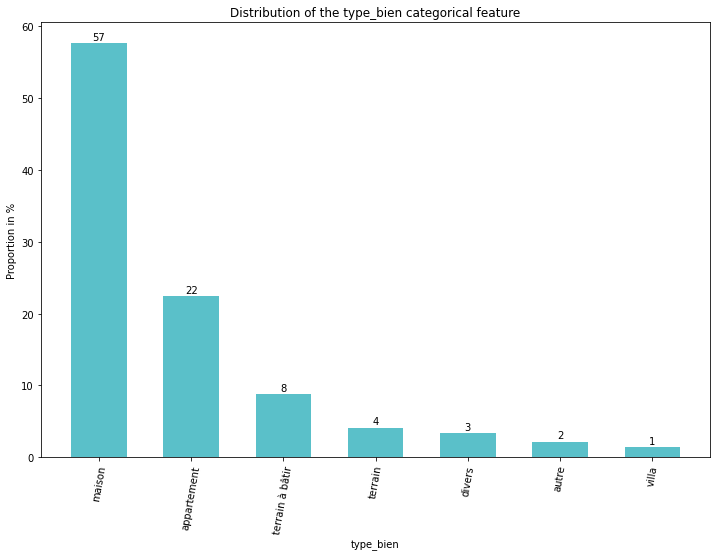Distribution of the property type variable before and after grouping least represented modalities
dummies = ['type_bien', 'type_client', 'source', 'exposition']
df = pd.get_dummies(df, columns=dummies)

Textual features

In the data that was scraped, there are two textual features, the title and the description. The description will be used to build another list price prediction model based on BERT in the next article. To make results comparable, it is best to use the same training and test observations. Outliers in the description feature will thus be dealt with right away.

Looking at the distribution of their lengths, we remark some incoherencies. As shown below, too short descriptions do not make much sense or do not actually describe the property.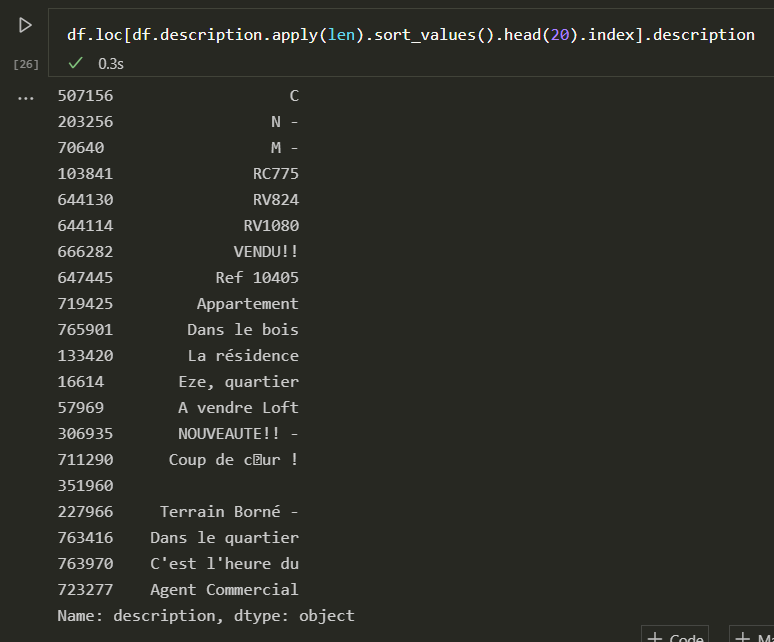Distribution of the length of the property descriptions in number of characters (left) and sample of the shortest descriptions (right)

Descriptions shorter than 100 characters are deemed to be uninformative and are excluded for the set.

df = df[df.description.apply(len)>100]

Multivariate analysis

At this point, we can consider our training set to be clean enough and ready for the actual modelling phase. Before diving into it, let’s do a quick multivariate analysis, looking at the Spearman correlations of the training features. Since we have many binary or discrete features in our data set, we chose to look at the Spearman correlation coefficient because it focuses on relative ranks rather than relative values like the Pearson coefficient does.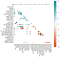Spearman correlation coefficients between feature, only displaying coefficients with an absolute value >0.2

As one could expect, the number of rooms, bedrooms, bathrooms… are positively correlated. Energy performance and greenhouse gas emission values and categories are also unsurprisingly positively correlated. Some property type indicator features are positively or negatively correlated with numerical features. Obviously, the “building plot” indicator is negatively correlated with the number of rooms, bathrooms… but interestingly, the “appartement” property type indicator is positively correlated with the longitude feature whereas it is negatively correlated with the “house” indicator suggesting that there may be more houses in the West and more apartements to the East. Further analyses would be needed to confirm this.

Pre-processing pipeline summary

Throughout the exploration phase of the training set, we constructed a pre-processing procedure that deduplicates the data, deals with missing values and outliers, selects and encodes the features.

We packed all these pre-processing steps into a scikit-learn pipeline module. For the sake of brevity, it is not included in this blog post.

71.5% of the original 300K observations are deemed pertinent for the modelling task. The training set thus stands at 215K observations of 66 features.

Supervised regression model

Our objective is to predict the list price of a real-estate listing based on the pre-processed features of our data set. The list price is a continuous numerical variable. We will thus chose a supervised regression model to predict it. In this article, we will focus on the numerical and categorical variables. The next post will show how the textual variable can be used for the same task.

To start off, both the training and test sets should be pre-processed and the features that are not to be considered in the model should be put aside.

from preprocessing import preprocessing_pipelinetrain_data = preprocessing_pipeline.fit_transform(train_data)
test_data = preprocessing_pipeline.transform(test_data)
train_data = train_data.drop(['titre', 'description','id_annonce',
'ville', 'departement'], axis = 1)
test_data = test_data.drop(['titre', 'description','id_annonce',
'ville', 'departement'], axis = 1)

We recommend choosing a gradient boosted tree supervised regression model for this price prediction task. We tried some linear regression methods such as Lasso, Ridge or ElasticNet but came to realise that they were outperformed by models such as XGBoost and Light GBM. Since LGBMs are faster to train, we stuck with that.

from lightgbm import LGBMRegressorestimator = LGBMRegressor(random_state = 42)

To rapidly tune the parameters of the LGBM model we use scikit-learn’s RandomizedSearchCV module. This method computes the cross-validated scores of the model for different combinations of parameters sampled randomly from a set or a distribution of possible parameters. It is less computationally costly than trying out all the possible combinations as is done with the GridSearchCV module yet it provides similar results when enough parameter combinations are taken.

from sklearn.model_selection import RandomizedSearchCVparameters = {
'num_leaves' : [10, 30, 50, 100, 200],
'max_depth': [None, 5, 10, 20, 50],
'n_estimators': [150, 200, 400, 600],
'learning_rate': [0.05, 0.1, 0.25, 0.5]
}
model = RandomizedSearchCV(estimator, parameters, random_state=42,
scoring = 'r2', n_iter = 50)

It took about 11 minutes to fit and tune the model, trying out 50 random combinations of the parameters listed above on CPU with 215K training observations and 5 fold cross validation.

X_train = train_data.drop(['prix'], axis = 1)
y_train = train_data.prix
model.fit(X_train, y_train)

Performance

Now that the list price prediction model is trained, it is time to evaluate it’s performance on the training set. To do so, we compute the price predictions for the pre-processed observations of the test set.

X_test = test_data.drop(['prix'], axis = 1)
y_test = test_data.prix
y_pred = model.best_estimator_.predict(X_test)

The scikit-learn library offers a variety of metrics to evaluate the performance of a supervised regression model. Most of the metrics summarise the distribution of the errors made by the model (MAE, MSE, MAPE…). It is interesting to look at these metrics alongside the R squared metric that quantifies the degree of any linear correlation between the predictions and true observed target values.

from sklearn.metrics import mean_absolute_error
from sklearn.metrics import median_absolute_error
from sklearn.metrics import mean_squared_error
from sklearn.metrics import mean_absolute_percentage_error
from sklearn.metrics import r2_score
mae = mean_absolute_error(y_test, y_pred)
mdae = median_absolute_error(y_test, y_pred)
mse = mean_squared_error(y_test, y_pred)
mape = mean_absolute_percentage_error(y_test, y_pred)
mdape = ((pd.Series(y_test.to_numpy()) \
- pd.Series(y_pred))\
/ pd.Series(y_test.to_numpy())).abs().median()
r_squared = r2_score(y_test, y_pred)

This model was able to predict the list price of the 311K properties from the separate test set averaging an absolute error of 62K euros with a median absolute error at 29K euros.
For this task, it makes sense to talk about error in relative terms, a 50K euro error on a million-euro property is not as bad as the same error on a 100K property. The Mean Average Percentage Error (MAPE) thus comes in handy. On average, the model predictions are off by 27% of the actual price but the Median Average Percentage Error is only 15%. The R squared score is also satisfactory, reaching 0.82.

Recommended next steps: explainability and robustness

An interesting complement to this study would be to explain how the model is actually predicting the list price of the property. A first step we can take in this direction is to look at the feature importance of the fitted LGBM.

feature_importances = \
pd.DataFrame(model.best_estimator_.feature_importances_,
index = X_train.columns, columns = ['importance'] \
).sort_values('importance', ascending=False)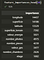Top 10 features by feature importance for the fitted LGBM model

With an LGBM, like other tree based ensemble learning models, the “importance” of each feature in the prediction decision can be measured as the decrease in the scoring metric at each split involving that feature weighted by the probability of reaching that split.

In the case of this model, the “feature importance” values suggest that the prediction value depends mostly on the location and size of the property which makes sense. Interestingly, the energy performance features and number of photos also seem to play a role. That said, conclusions based on feature importance values are to be taken with a pinch of salt, they can be misleading. When features are correlated, their features importance value is shared between them, thus understating their potential predictive power.

A more sophisticated and robust method to analyse the influence of features could be to look at SHAP values. They can provide information about both the magnitude and direction of the value of a feature can have on the final prediction.

It would also be interesting to perform some robustness tests, comparing performances of our model for different housing types, for different areas, different countries… We could also test our model for robustness in time. How would this model perform on listings that are several years old or on future listings?

Conclusion

In this article we explored real-estate data and constructed a model that is capable of predicting the list price of a real-estate property based on a set of numerical and categorical features scraped from online listings. The performance we got out of this model is satisfactory, it is a lot better than random but there remains room for improvement.

In our next post, we will exploit the textual information contained in the description of the real-estate property to predict the list price. We will see if we can leverage the power of NLP to achieve similar if not better results than those we got today.

The purpose of the second article is to provide a practical example of how Google’s BERT can be fine-tuned for a regression task. Usually most posts focus on fine-tuning BERT for classification, but we will be showing that it can easily be adapted to regression tasks too.

Acknowledgements

I would like to give a special thanks to Kawtar Zaher, Anne-Marie Heng, Lorraine Hickson and Louis Boulanger for their great contribution to the this article.